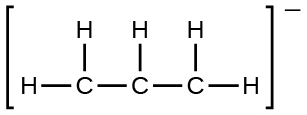Problem: Write resonance forms that describe the distribution of electrons in each of these molecules or ions.the allyl ion:

FREE Expert Solution

We are being asked to identify the resonance forms that describe the distribution of electrons in allyl anion (C3H5-).

We will have to draw the Lewis Structure of C3H5- first.

Step 1: Determine the central atom in this molecule.

•  H is less electronegative than C but H can only make one bond
•  C are the
central atoms and is bonded to the H atoms

Step 2: Calculate the total number of valence electrons present.

C3H5-:

Group              Valence Electrons

C          4A                    4 valence e x 3
H          1A                    1 valence e x 5
= 17 valence e

-1 charge                     +1 e­-

Total = 18 valence e-

Step 3: Draw the Lewis Structure for the molecule

•  C → prefers to have a complete octet (8 e- around them)

91% (402 ratings)
Problem Details

Write resonance forms that describe the distribution of electrons in each of these molecules or ions.

the allyl ion: